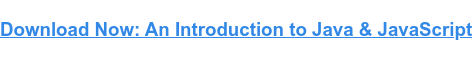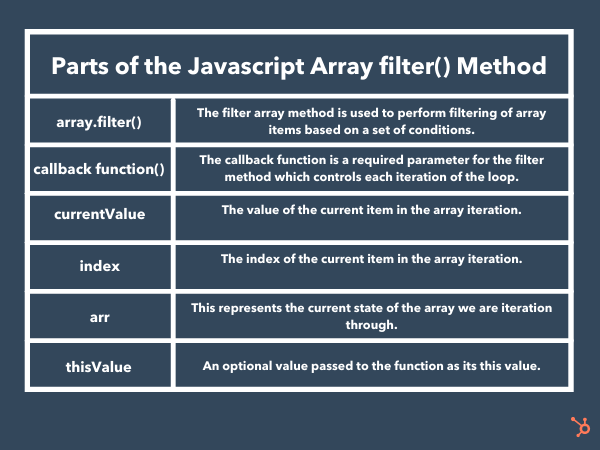# JavaScript filter Array Method: An Introductory GuideUpdated:

Published:

Like Java and filters, JavaScript and array methods go hand in hand. Without array methods, the functionality of JavaScript would be severely reduced. We'd have an array of arrays and nothing to do with them.With that in mind, in this post, we will be discussing the benefits of the JavaScript array method known as filter(), which filters data from an array. First, we will be discussing what the filter() method is and how it works, after which we will look at some examples of it in action.

Without further delay, let's jump right in.## JavaScript filter

JavaScript arrays are one of the most powerful data structures that JS offers, and it comes with some methods that can bolster its functionality. The JS filter() method is an excellent example of the powerful methods provided.

The filter() method provides a means to filter data from an array based on certain conditions. It works by taking all values from a provided array that meets set criteria and entering them into a new array.

The process sounds simple and often is, but its power is seen best in more complex tasks. Let's look at how the JS filter() method works, its syntax, and some examples in action.

## What does the filter() method look like?

In many ways, the JavaScript filter() method looks like any other method, except for the parameters it accepts. The filter method — like many methods — accepts parameters that provide more options for how we can manipulate the data within an array. Let's look at the syntax for this method and break it down into manageable steps.Let’s dissect this syntax into parts, starting with the method syntax without its parameters.

### JavaScript filter() Syntax

The syntax here is simple, and you call the filter method on the array you want to use it on. In the example above, array is the target, and .filter() is the method called on it.

` `array.filter()``

The filter method acts as an iterator, looping through the array one item at a time, stopping when the array ends. Next, let's look at the parameters that this method can accept.

### The array.filter() Function Parameter

The filter() method accepts two parameters; the first is a function that will run against the array. Developers use this function to create everything from simple conditional statements to complex equations. This function accepts three parameters; only the first is required.

` `function(currentValue, index, arr)``

The function has access to the array through the method. The currentValue parameter inside the function holds the array item that the filter method is currently accessing. Removing the optional parameters, we can see a basic example of how this works.

Assuming the following array of numbers

` `const nums = [1, 2, 3, 4, 5, 6, 7];``

Using ArrWe could run a function to test if any of the values are odd or even. The filter will then assign all numbers that meet the check to a new array.

` `let evenNums = nums.filter( num => !( num % 2) );``

The above arrow function would successfully return any array item that is an even number, and then the filter method appends it to a new array object. The variable evenNums would then contain an array of even numbers from the original array.

` `[2,4,6]``

In the case of more complex equations and actions, you might declare a named function and reference the function by name.

Let's look at that next, assuming the same array and goal as above.

` `/* to get odd numbers simply remove the !*/nums.filter( evenNums );let evenNums = num => { If ( !(num % 2) ) { }};``

Please note that there are caveats to consider when deciding to use a traditional function declaration or an arrow function declaration. It’s important to consider hoisting needs, closure, use of this keyword, and if you'll need to create new instances.

Now that we have covered the mandatory parameters needed for this method, let's consider how to incorporate and use the optional parameters.

## How to Use Filter in JavaScript

Since the other parameters are optional, we can briefly go over them and cover some examples. Seeing all available parameters in action will help illuminate how they work.

` `nums.filter(function(num, index, arr), thisValue);``

The index parameter of the function contains the index location of the current item in the iteration, starting at 0 incrementing with each iteration. The index is accessible from within the function, and this gives developers more control over how they access the items in the array and allows for accurate manipulation.

The same is true of the arr parameter; accessing it within the function allows developers to monitor the current state of the array as the filter method progresses through iterations. Finally, the last parameter for the filter method is passed as the this value of the method. The this value of the method contains any data you wish to be contextually relevant to the operation.

Again, this is a lot to drink in, so let's look at how to use these parameters in practice and solidify how they work.

### JavaScript filter() Example

In this example, instead of checking for even or odd values, we will look at how to set a range for our numbers. Furthermore, using console.log(), we can observe the index of each iteration and the current state of the array.

` `let nums= [ 50, 40, 10, 16, 12 ];let range = { bottom: 45, top: 100};let teenagers = people.filter(function (person, id, arr) {console.log( arr[id] )arr[id] = arr[id] + arr[id]return person >= this.lower && person <= this.upper;}, range)``

Running the above code would log the id of the current iteration, then change the value of each item by adding its value to itself. So essentially, it would double each value and then add each value within the range to a new array.

## Using the JavaScript filter() method

We have discussed the properties of the filter method and all of the parameters. We've even seen a couple of examples of how it works. By now, you have been introduced to the core concepts behind it. Let's solidify that knowledge by briefly going over these concepts.

function(currentValue, index, arr), thisValue

• array.filter(): Iterates over an array, once for each item, and creates a new array based on results from given parameters. It accepts two parameters, and the first is a required function. The second is used to provide context under which the function should behave.
• function(currentValue, index, arr): Requires a function parameter to run. The function manipulates how the array items are handled and added to the new array.
• thisValue: The method accepts an optional second parameter. This parameter is used to create a this value, which can be accessed during the function's operation. This context can then be used to further control what information is added to the new array.
• currentValue, index, arr: The function parameter takes three parameters, the first required and holds the current item in the array. The other two are optional, one to count the current index of the array item and one to view the current state of the array.Topics: Javascript

## Related Articles

We're committed to your privacy. HubSpot uses the information you provide to us to contact you about our relevant content, products, and services. You may unsubscribe from these communications at any time. For more information, check out our Privacy Policy.